Publicité

# MATH 7-W7.pptx

2 Apr 2023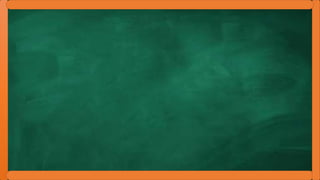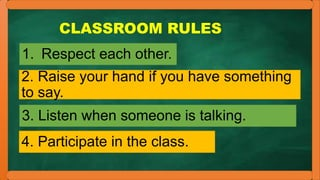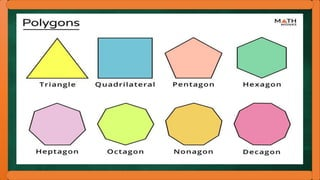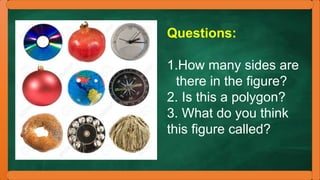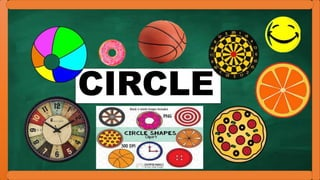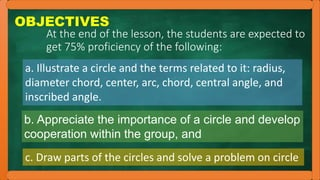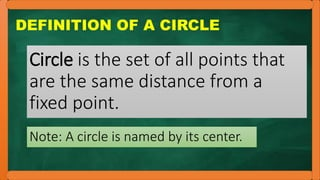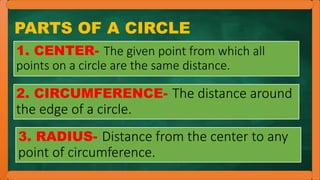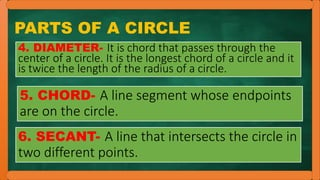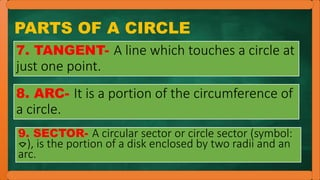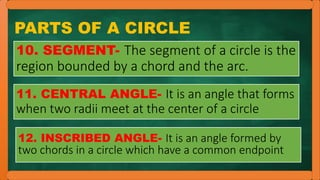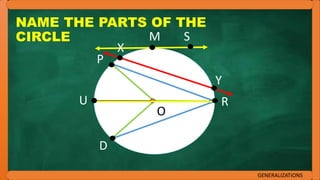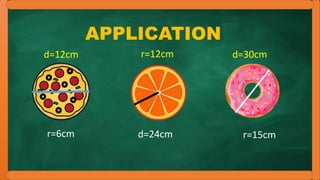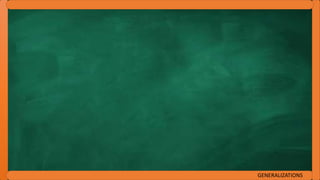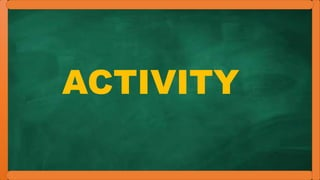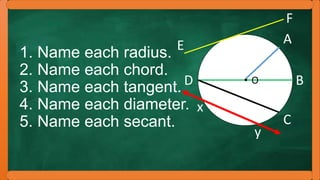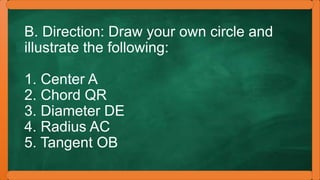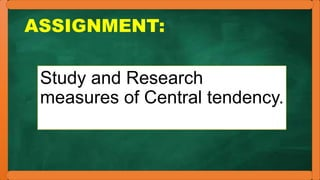1 sur 21
Publicité

### MATH 7-W7.pptx

1. CLASSROOM RULES 1. Respect each other. 2. Raise your hand if you have something to say. 3. Listen when someone is talking. 4. Participate in the class.
2. Questions: 1.How many sides are there in the figure? 2. Is this a polygon? 3. What do you think this figure called?
3. CIRCLE
4. OBJECTIVES At the end of the lesson, the students are expected to get 75% proficiency of the following: a. Illustrate a circle and the terms related to it: radius, diameter chord, center, arc, chord, central angle, and inscribed angle. b. Appreciate the importance of a circle and develop cooperation within the group, and c. Draw parts of the circles and solve a problem on circle
5. DEFINITION OF A CIRCLE Note: A circle is named by its center. Circle is the set of all points that are the same distance from a fixed point.
6. PARTS OF A CIRCLE 1. CENTER- The given point from which all points on a circle are the same distance. 2. CIRCUMFERENCE- The distance around the edge of a circle. 3. RADIUS- Distance from the center to any point of circumference.
7. PARTS OF A CIRCLE 5. CHORD- A line segment whose endpoints are on the circle. 4. DIAMETER- It is chord that passes through the center of a circle. It is the longest chord of a circle and it is twice the length of the radius of a circle. 6. SECANT- A line that intersects the circle in two different points.
8. PARTS OF A CIRCLE 7. TANGENT- A line which touches a circle at just one point. 8. ARC- It is a portion of the circumference of a circle. 9. SECTOR- A circular sector or circle sector (symbol: ⌔), is the portion of a disk enclosed by two radii and an arc.
9. PARTS OF A CIRCLE 10. SEGMENT- The segment of a circle is the region bounded by a chord and the arc. 11. CENTRAL ANGLE- It is an angle that forms when two radii meet at the center of a circle 12. INSCRIBED ANGLE- It is an angle formed by two chords in a circle which have a common endpoint
10. NAME THE PARTS OF THE CIRCLE GENERALIZATIONS P M O R D U X Y S
11. APPLICATION r=6cm r=12cm d=30cm d=24cm r=15cm d=12cm
12. GENERALIZATIONS
13. VALUES INTEGRATION
14. ACTIVITY
15. 1. Name each radius. 2. Name each chord. 3. Name each tangent. 4. Name each diameter. 5. Name each secant. • O A B C D F E y x
16. B. Direction: Draw your own circle and illustrate the following: 1. Center A 2. Chord QR 3. Diameter DE 4. Radius AC 5. Tangent OB
17. ASSIGNMENT: Study and Research measures of Central tendency.
18. THANK YOU FOR LISTENING! GOD BLESS! 
Publicité